Our World Statistics Day conversations have been a great reminder of how much statistics can inform our lives. Do you have an example of how statistics has made a difference in your life? Share your story with the Community!
Choose Language Hide Translation Bar
Alias Optimal versus D-optimal designs

In my previous blog entry, I discussed the purpose of the Alias Matrix in quantifying the potential bias in estimated effects due to the alias terms. In this blog post, we look at an example that creates a D-optimal design and an Alias Optimal design with the same number of factors and runs.

Consider an experiment where we have six two-level categorical factors denoted by X1 to X6, and a budget of 12 runs. Our interest is in the main effects.

We start with the D-optimal design. Using the Custom Designer with six factors and 12 runs for the main effects, JMP will find a D-optimal design by default. If we take a look at the Color Map On Correlations below, we see all the main effects are orthogonal to each other, but correlated with two-factor interactions (absolute correlation of 0.3333). Since our model contains only main effects, looking at the design diagnostics and estimation efficiencies reveal that we are not going to find a better design for getting precise estimates of the effects in this model.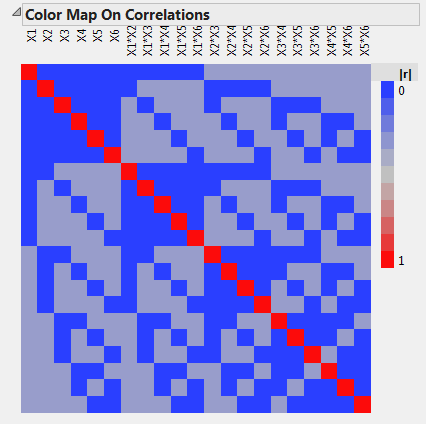Taking a look at the Alias Matrix, we find the following: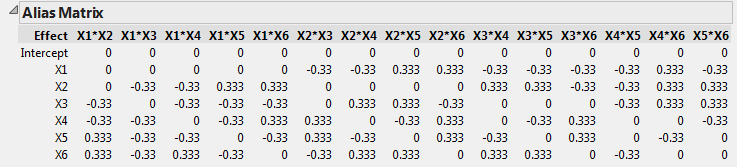The entries in the Alias Matrix and Color Map On Correlations do not generally correspond directly to each other as they do in this example. If you try this out on your own, you may notice different positions for the positive and negative values in the Alias Matrix.

Recalling the discussion in my previous blog entry and examining the row of the Alias Matrix for X1, we see that the estimate for the main effect for X1 is biased by any two-factor interaction not involving X1. For example, say that the main effect for X1, $$\\beta_1 = 3$$, while the X2*X3 interaction and X2*X4 interaction are non-zero, with $$\\beta_{23}=2$$ and $$\\beta_{24}=4$$ respectively. The expected value for the estimate of $$\\beta_1$$, given by $$\\hat{\\beta_1}$$ is then:

$$\\begin{eqnarray*} E(\\hat{\\beta_1}) &= &\\beta_1 - 0.33 * \\beta_{23} - 0.33 * \\beta_{24} \\newline &=& 3 - 0.33*2 - 0.33*4 \\newline &=& 1. \\end{eqnarray*}$$

The expected value for the estimated main effect for X1 is much smaller than its true value, which means it is likely the effect will appear less important than it is when running the experiment. Conversely, there are also cases where this aliasing can make the expected value for an effect much larger than its true value.

What if I want to minimize this bias?

Surely, if there is a blog post on this topic, there must be a way to deal with this. In Custom Design, before hitting the “Make Design” button, if you take a look under the red triangle, you’ll notice a few different options under Optimality Criterion. What we want is to select “Make Alias Optimal Design.”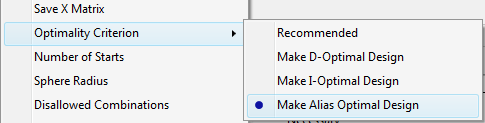Setting up the same 12-run design with the Alias Optimal criterion, we get the following Alias Matrix: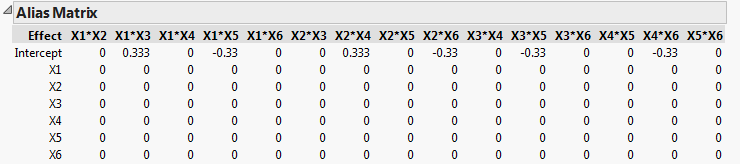In this case, only the estimate of the intercept is biased by two-factor interactions. Our main effect estimates are unbiased from the potentially active two-factor interactions. For the bias we see in regards to the intercept, this is less of a concern since we are typically less interested in a precise estimate of the intercept, but rather identifying which factors are important.

However, there is a cost for choosing alias optimality. Examining the Color Map On Correlations, we see that the main effects are not all orthogonal. Whereas the D-optimal design was 100% D-efficient, the alias optimal design has a D-efficiency of around 92%. Examining the Estimation Efficiencies tab, we see the confidence intervals for the main effects are about 10% longer compared to the D-optimal design. If the bias from the alias terms is a concern, this seems like a small price to pay.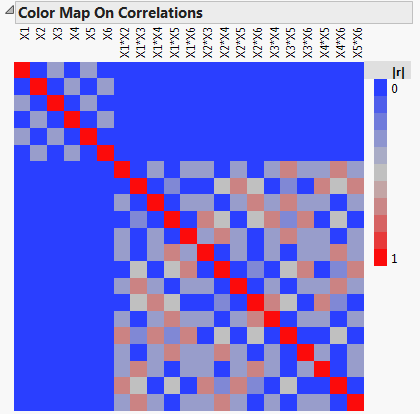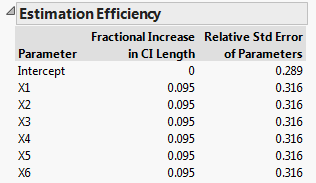Which design should I use?

The choice between the two designs depends on how concerned you are about the number and size of active two-factor interactions. The D-optimal design gives more precise estimates of the main effects, but you run the risk of biased estimates if two-factor interactions are important. The Alias Optimal design protects from this bias for a small reduction in the precision of the estimates.

If

1. all the factors have two-levels as in many screening designs, and
2. you can afford an even number of runs that is at least twice the number of factors,
3. then an Alias Optimal design can remove all the potential bias of main effects due to two-factor interactions. This makes Alias Optimal designs less risky for non-expert practitioners than either Resolution III fractional factorial designs or Plackett-Burman designs. You can find more details on Alias Optimal designs in Jones and Nachtsheim (2011).

Jones, B. and Nachtsheim, C. J., (2011) “Efficient Designs with Minimal Aliasing” Technometrics 53:62-71.

Article Labels

There are no labels assigned to this post.

Article Tags
Visitor

Jed Campbell wrote:

This was a very clear explanation. Thanks!

If you could afford 1 more run, would a Definitive Screening Design be a better choice? As I understand, there would be no correlation between the main factors and any 2 way interactions, and the Alias Matrix would show zeroes all the way across. It might be nice to have a "Part 3" focusing on this idea. Thoughts?Staff

Ryan Lekivetz wrote:

Great question!

In fact, Definitive Screening Designs are a special case of Alias Optimal Designs, where the main effects are orthogonal to not only the two-factor interactions, but also the quadratic effects. They also allow estimation of the quadratic effects. Assuming that my factors were continuous and I had any notion there was curvature, I would go with the Definitive Screening Design. You lose a little bit of D-efficiency compared to the Alias Optimal Design, but the orthogonal main effects in the Definitive Screening Design means the diagnostics are comparable.

If I'm dealing with 2-level factors only, I like to use the Custom Design platform since I have some flexibility with the D-efficiency weight under advanced options.

Thanks for the input and suggestion, and for interested readers, there is an original blog post on definitive screening designs.Super User

Some of the mark-up is not rendering correctly.